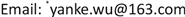K-means聚类分析，KNN算法，协同过滤，个性化推荐, K-means KNN Collaborative Filtering Personalized Recommendation1. 引言

2. 协同过滤算法

1) 建立用户模型

2) 寻找目标用户的邻居

S i m ( i , j ) = ∑ q = 1 15 ( R i , q − R i ¯ ) ( R j , q − R j ¯ ) ∑ q = 1 15 ( R i , q − R i ¯ ) R j ¯ ∑ q = 1 15 ( R j , q − R j ¯ ) 2 (1)

3) 产生目标用户的推荐产品

P i , q = R i ¯ + ∑ j ∈ U s i m ( i , j ) × ( R j , q − R j ¯ ) ∑ j ∈ U ( | s i m ( i , j ) | ) (2)

3. 数据来源及预处理

1) 去除回看信息重复和用户点播信息重复的数据、观看时间为空的记录和观看时长小于一分钟的记录。

2) 将其中冗余的属性以及与挖掘过程不相关的属性剔除得到处理后的数据，如表1所示。

Form of data processin

06月28日 自然：猫——隐匿的野性科学教育52:36.
.........

4. 建立用户偏好模型4.1. 构建用户偏好类型

Matrix: “user-type-duration

1000115.5000...0
100021396.44.2263.0300.4...72.2
10003183.84.1021.9...26.0
.....................
11329249.90.30753.1...24.9

4.2. 用户类型的偏好度

i n t e r e s t ( i , q ) = ∑ k = 1 n   t l a b e l ( i , q ) [ k ] ∑ q = 1 m ∑ k = 1 n   t l a b e l ( i , q ) [ k ] (3)

L o y a l t y ( i , q ) = ∑ k = 1 n   t l a b e l ( i , q ) [ k ] ∑ k = 1 n   t p r o g r a m ( i , q ) [ k ] (4)

L a b e l ( i , q ) = ω 1 L o y a l t y ( i , q ) + ω 2 i n t e r e s t ( i , q ) (5)

These preference types for each use

10001电视剧新闻综艺
10002电视剧少儿动画综艺
......
11329电视剧新闻电影

5. 协同过滤算法对单个用户的推荐5.1. 建立用户模型

5.2. 寻找目标用户的邻居

5.3. 产生目标用户的推荐类型

5.4. 实现用户的节目推荐

Recommended program for individual use

10001琅琊榜1.00
10001西游记续大闹披香殿加防抖1.00
10001白夜追凶0.99
10002西游记续大闹披香殿加防抖1.00
10002秦时明月0.97
.........
11329百鸟朝凤0.93
11329碟中谍神秘国度调整字幕0.92

6. 用户的打包推荐6.1. K-means聚类分析与KNN算法进行用户分群

Clustering center of K-means algorith

11156.329.0304.1210.031.6
22900.2123.83091.5974.568.1
994427.7225.25302.3618.545.7
1009520.2310.52950.11475.922.0

User groups of individual use

1000168
1000225
1132824
1132987

Preference types of each user grou

1电视剧综艺新闻
2少儿动画电视剧体育
............
99体育电视剧综艺
100电视剧电影新闻

6.2. 节目产品的打包和推送

Recommended program package for each user grou

1琅琊榜
1广视新闻524.4
2艾可魔法少女
2国际田联砖石联赛奥斯陆站482.3
100琅琊榜
100广视新闻679.7

7. 结论及展望

1) 各个电视台的节目类型时间比例是根据各个电视台(分周一至周五和周末)的常规播放规律来进行计算的，与准确的节目类型播放规律会有一定的误差。

2) 评分较高的节目推荐给目标用户，往往会导致推送的不精准和有一大部分评分较低的节目没有用户去收看。